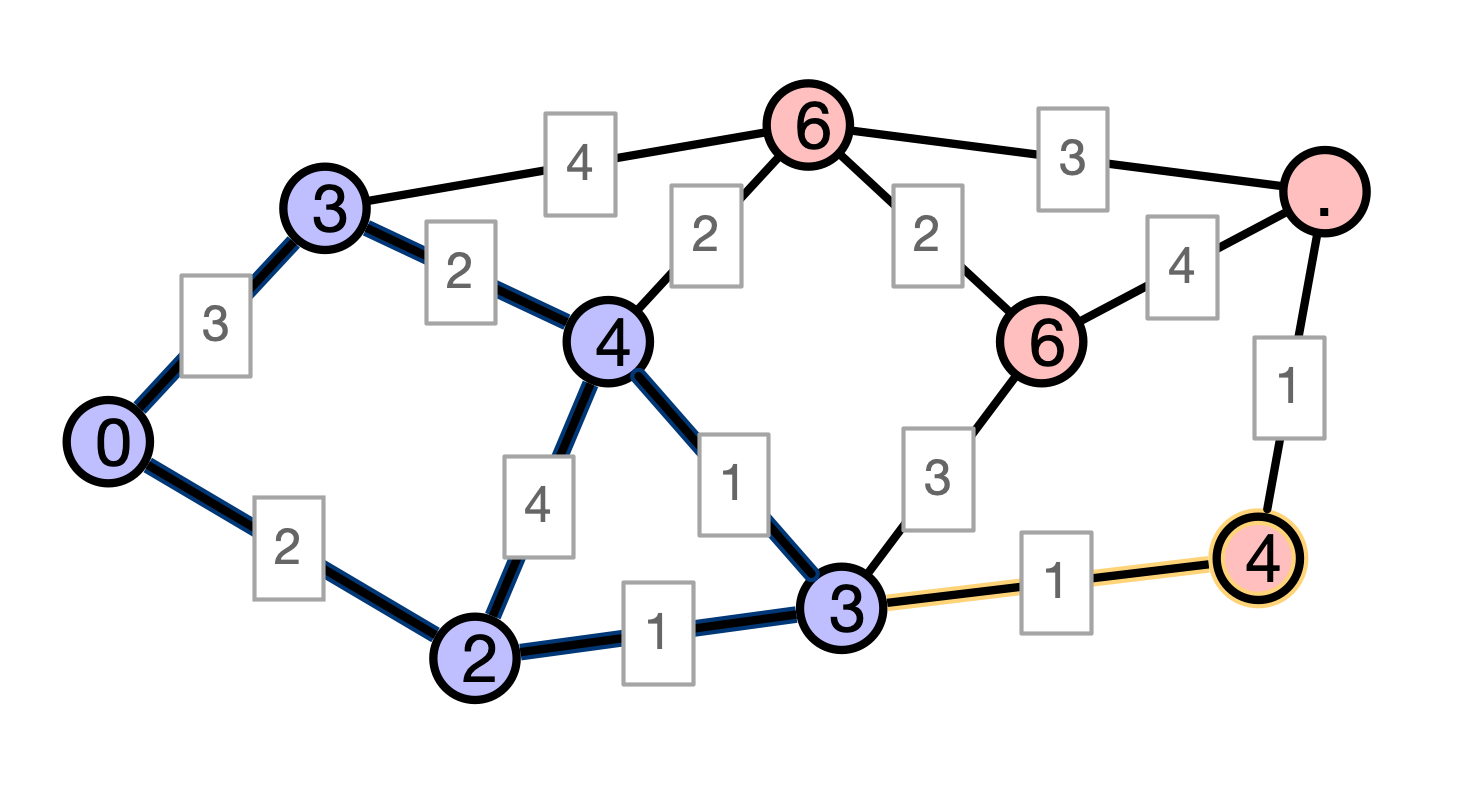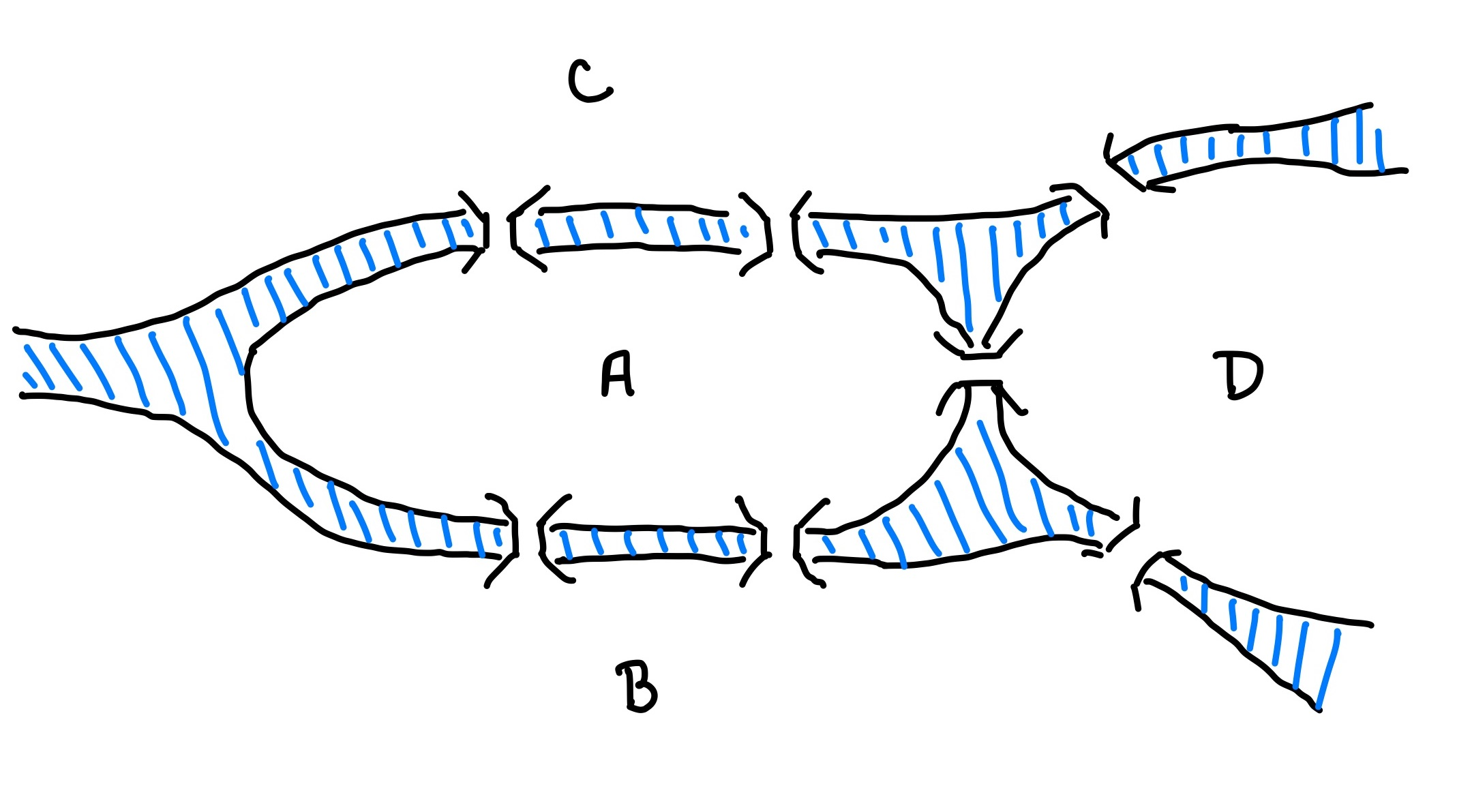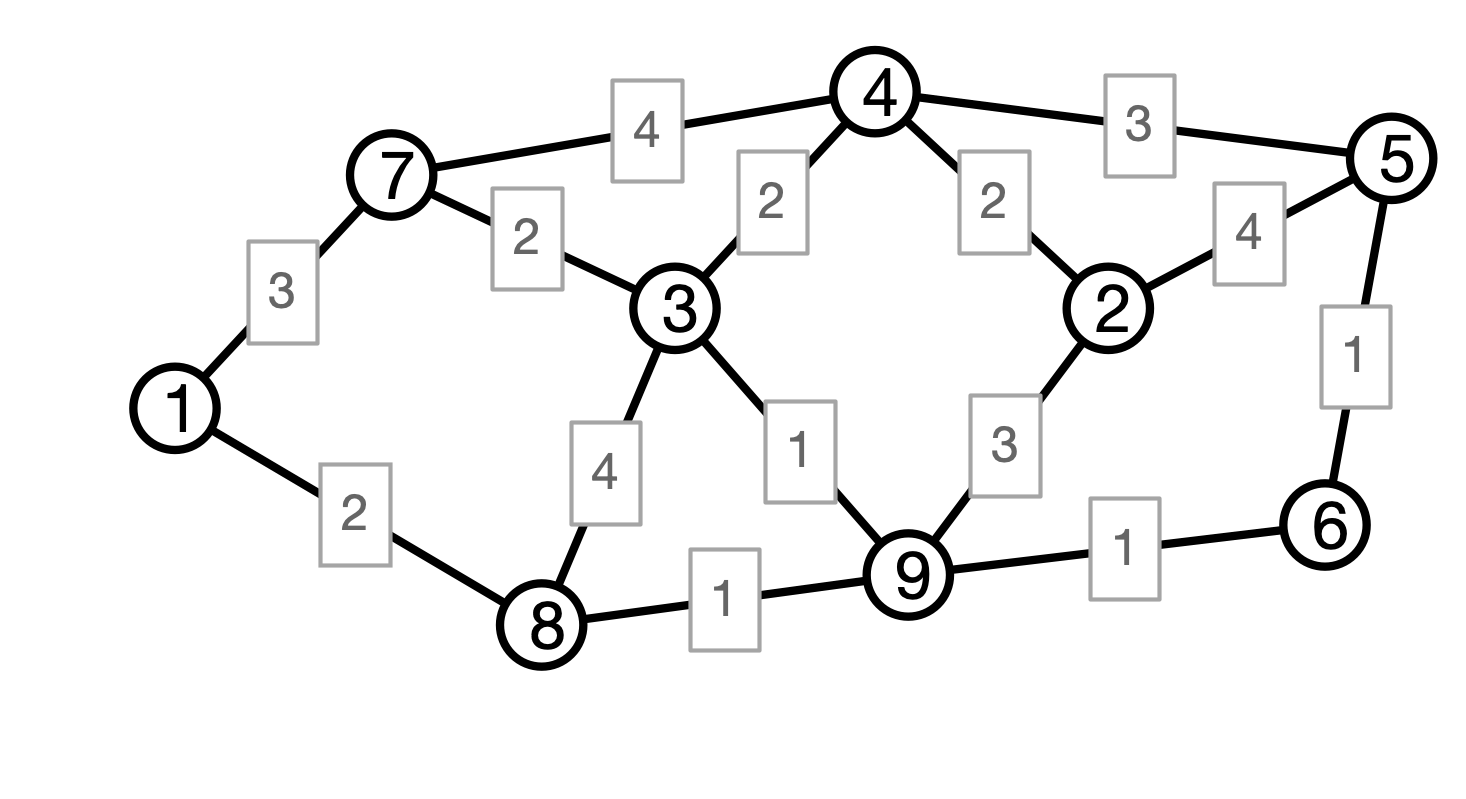# Lecture 17: Dijkstra and Minimum Spanning Trees

### COSC 311 Algorithms, Fall 2022

$\def\compare{ {\mathrm{compare}} } \def\swap{ {\mathrm{swap}} } \def\sort{ {\mathrm{sort}} } \def\insert{ {\mathrm{insert}} } \def\true{ {\mathrm{true}} } \def\false{ {\mathrm{false}} } \def\BubbleSort{ {\mathrm{BubbleSort}} } \def\SelectionSort{ {\mathrm{SelectionSort}} } \def\Merge{ {\mathrm{Merge}} } \def\MergeSort{ {\mathrm{MergeSort}} } \def\QuickSort{ {\mathrm{QuickSort}} } \def\Split{ {\mathrm{Split}} } \def\Multiply{ {\mathrm{Multiply}} } \def\Add{ {\mathrm{Add}} } \def\cur{ {\mathrm{cur}} } \def\gets{ {\leftarrow} }$

## Overview

1. Dijkstra’s Algorithm Correctness
2. Implementing Dijkstra
3. Minimum Spanning Trees

## Last Time: Weighted SSSP

Input.

• a weighted Graph $G = (V, E)$, edge weights $w$
• an initial vertex $u \in V$
• each vertex $v \in V$ has associated adjacency list
• list of $v$’s neighbors
• includes weight of edge from $v$ to each neighbor

Output.

• A map $d: V \to \mathbf{R}$ such that $d[v] = d_w(u, v)$ is the graph distance from $u$ to $v$

• $d[v] = \infty$ indicates no path from $u$ to $v$

## Dijkstra’s Algorithm

1. Initialize $d[u] = 0$ and $d[v] = \infty$ for all $v \neq u$
2. Maintain set $S$ of finalized nodes, initially empty
3. Process nodes. While $S \neq V$ do:
• find node $v$ in $V - S$ with minimal $d[v]$
• add $v$ to $S$
• for each neighbor $x$ of $v$
• update $d[x] \gets \min (d[x], d[v] + w(v, x))$

## Correctness

1. Initialize $d[u] \gets 0$ and $d[v] \gets \infty$ for all $v \neq u$
2. Maintain set $S$ of finalized nodes, initially empty
3. Process nodes: while $S \neq V$
• find node $v$ in $V - S$ with minimal $d[v]$
• add $v$ to $S$
• for each neighbor $x$ of $v$
• update $d[x] \gets \min (d[x], d[v] + w(v, x))$

Claim. For every vertex $v \in S$, $d[v]$ stores the correct (weighted) distance $d_w(u, v)$.

## Proof of Claim

Claim. For every vertex $v \in S$, $d[v]$ stores the correct (weighted) distance $d_w(u, v)$.

Proof. Use induction on size of $S$. Set $k =$ size of $S$.

Base case $k = 1$. Only $u$ is added to $S$. Set $d[u] \gets 0$, which is correct answer.

## Inductive Step I

Inductive hypothesis. When $S$ contains $k$ elements, $d[v]$ is correct for all vertices $v \in S$.

Consider next iteration of outer loop:

• $x$ has $d[x] = \min_{v \in S} (d[v] + w(v, x))$## Inductive Step II

Must show: $d[x] = d_w(u, x)$; argue by contradiction

1. suppose $d[x] \neq d_w(u, x)$
2. observe: there is a path from $u$ to $x$ of length $d[x]$
3. $\implies d_w(u, x) < d[x]$
4. $\implies$ there is a path $P$ from $u$ to $x$ of length $\ell < d[x]$## Shorter Path Illustration## Inductive Step III

Must show: $d[x] = d_w(u, x)$; argue by contradiction

1. suppose $d[x] \neq d_w(u, x)$
2. observe: there is a path from $u$ to $x$ of length $d[x]$
3. $\implies d_w(u, x) < d[x]$
4. $\implies$ there is a path $P$ from $u$ to $x$ of length $\ell < d[x]$
5. $P$ must leave $S$ at some point $y$
6. by definition of $x$, any path from $u$ to $y$ must be longer than $d[x]$
7. $\implies w(P) \geq d[x]$, which contradicts $4$

Conclusion. $d[x] = d_w(u, x)$, as claimed.

## Dijkstra Running Time?

$G$ has $n$ vertices, $m$ edges

Question. How many operations performed?

1. Initialize $d[u] \gets 0$ and $d[v] \gets \infty$ for all $v \neq u$
2. Maintain set $S$ of finalized nodes, initially empty
3. Process nodes: while $S \neq V$
• find node $v$ in $V - S$ with minimal $d[v]$
• add $v$ to $S$
• for each neighbor $x$ of $v$
• update $d[x] \gets \min (d[x], d[v] + w(v, x))$

## For Simplicity

Assume. Vertices are $1, 2, \ldots, n$

• $d$ is an array with $d[v] =$ distance from $u$ to $v$
• $\mathrm{final}$ is a Boolean array with $\mathrm{final}[v] = \mathrm{true}$ if $v$’s distance is finalized
• keep track of number of finalized vertices
• we’re done when $n$ vertices are finalized

## Simple Implementation

For step

• find node $v$ in $V - S$ with minimal $d[v]$

use linear search

Question 1. What is running time of finding min?

Question 2. What is overall running time of Dijkstra?

## Faster Implementation?

Since we need to access $v$ with minimum $d[v]$, store non-finalized vertices in a priority queue

• store elements with associated priorities
• add element with given priority
• remove element with smallest priority

Heap priority queue implementation

• supports these operations with running time $O(\log n)$

For Dijkstra:

• Store un-finalized vertices in priority queue
• priority of $v$ is $d[v]$

## One Sublety, Two Solutions

Issue. Dijkstra decreases priority of vertices

Solution 1. Store duplicate vertices with each new distance

• will still find vertex $v$ with smallest $d[v]$
• if finalized vertex is returned, ignore it
• requires priority queue of size $m$ rather than $n$

Solution 2. Use more sophisticated priority queue that supports “decrease priority” operation

• can be implemented in $O(\log n)$ time

## Conclusion

Dijkstra performs

• $n$ removals of vertices when they are finalized
• $2m$ distance updates

With efficient priority queues, these operations can each be performed in $O(\log n)$ time so…

Result. Dijkstra’s algorithm can be implemented to run in time $O(m \log n)$.

## DPW in Königsberg

Winter is coming to Königsberg!

• Must prepare the bridges for ice
• Each bridge has an associated cost to de-ice
• Königsberg doesn’t have the budget to de-ice all bridges
• For public safety, must ensure that all landmasses are reachable from each other

Question. How to find the cheapest set of bridges to de-ice that maintain connectivity?

## Picture## Graph Problem

Input:

• a weighted graph $G = (V, E)$ with edge weights $w$

Output:

• a set $F$ of edges in $E$ such that

1. $(V, F)$ is connected
2. sum of weights of edges in $F$ is minimal among all connected sub-graphs of $G$

The graph $T = (V, F)$ is called a minimum spanning tree of $G$

## Example## Next Time

Greedy algorithms for the minimum spanning tree problem!